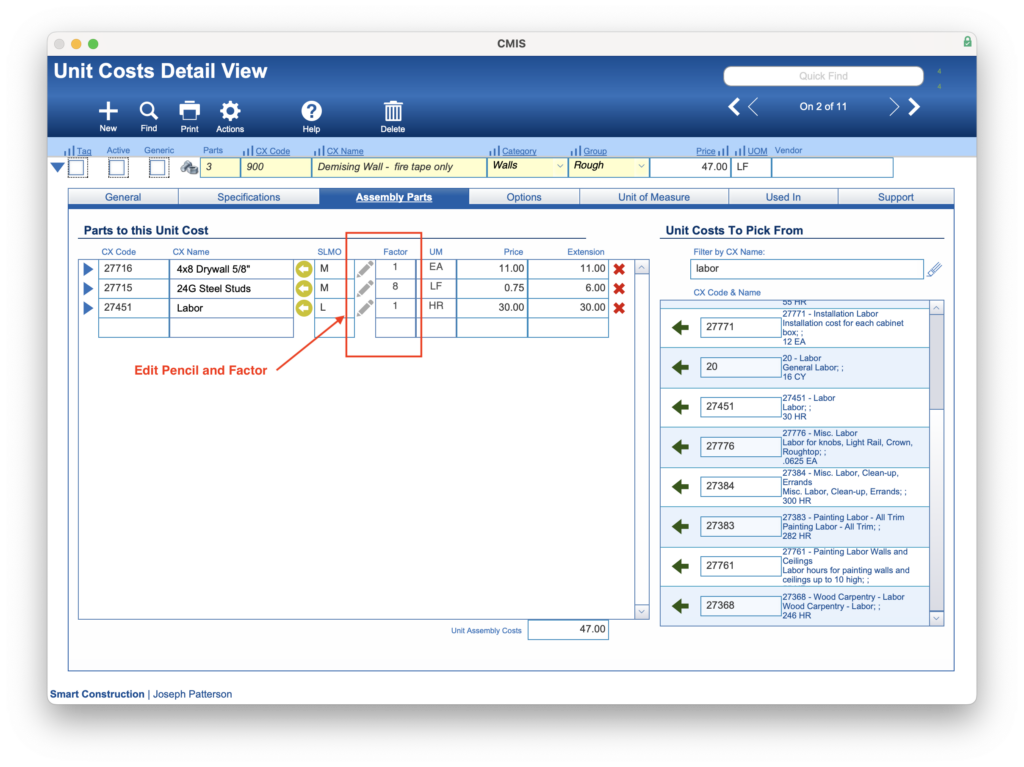# Assembly Part Factors – Adding Dynamic Factor Formulas

### Guide Steps

#### Introduction / Overview

A CMIS Assembly is a Unit Cost that is made up of other unit cost items as its assembly parts.  And these parts can have factors.  A factor is used to determine the quantity of the parts needed for the Assembly.  A key new feature of “factors” is that they can have their own “formulas” that can be modified at the time of the estimate by Plan Quantity Variables or Nums.

This guide will walk you through the steps to help properly create a formula that can dynamically modify the factor based on Plan Quantity values.

What you will need to know:

• You should be familiar with the concept of Plan Quantities.
• You should be familiar with using Unit Costs.
• You should have some working knowledge of how FileMaker Pro formulas work.
1

#### Go to the Edit Screen for a Factor Formula / Note

To get to the screen to enter or edit a Factor Formula, follow these steps:

1. Navigate to Estimating > Unit Cost > Detail View > Assembly Parts for the Unit Cost assembly you want to work on.2. Click on the Pencil icon to open the Assembly Parts window:2

While in the Assembly Parts window, you’ll notice three main areas. 1) The name of the assembly and its part; 2) The Note or Formula field; and 3) The Variables screen (NOTE: that this can switch between Variables and Nums)

In the example shown, we are building an assembly of a Demising wall for office space in a commercial project.  Under the Variables Used in Quantity Templates, we have filtered those variables to only those on our TIQ1 (TI1 Quantity Template).   We can see that we have a variable “HTDW,” which is for the Demising Wall Height.  This would be used in our project Plan Quantity area to identify how high we would like a specific demising wall to be.  (The “LFDW” variable would be used to identify the linear footage of the wall that is needed)

Since the Assembly is based on (or looking for a quantity of), Lineal Footage of the wall and this part (4×8 sheets of drywall) is based on sheets; we need to write a formula that can determine how much of a sheet of drywall is needed for one foot of the wall.

In this case, that formula is:

`!HTDW! * 2 / 32`

We wrap a variable in exclamation points (!) to signify to CMIS that this is a Plan Quantity variable.  Therefore !HTDW! will get replaced with the value that was entered for the HTDW variable (in the example, 16 feet), then it will be multiplied by 2 (for both sides of the demising wall), and that total will be divided by 32 (the number of square feet in a sheet of drywall).  The answer will be 1 sheet of drywall will be needed per lineal foot of a 16′ high demising wall.

Another way to write this formula utilizing “//” notes to better document how this formula was designed would be:

```Let ( [ ~height   = !HTDW! ; //The height of the demising wall
~sides    = 2 ;      //Drywalled both sides
~perSheet = 32       //The number of Square Feet per 4X8 Sheet of Drywall
] ;

( ~height * ~sides ) / ~perSheet

)```
3

#### Test the Formula

Once the formula is written, you can test it by clicking the Gears under the Factor and above the Formula fields.

Clicking this Gears button will utilize the Sample values that were entered to determine what factor to use for the assembly.  In our example, we used a Sample value of 16, which resulted in 1 sheet.

Always try different sample values to verify that your formula makes sense and is calculating correctly.

4

#### How Factor Formulas Perform in an Estimate

Using Assembly Unit Costs with Factor Formulas in an estimate, there are a few steps that need to be made:

1. Drill down on an Estimate Line Item so that you are on the Estimate Level 1 screen.
2. Add the assembly parts to all estimate lines.  Do this by selecting “Add Assembly Parts to All Line items” from Actions.
3. Then select “Calculate Quantities (Found Set)” from Actions.

5